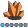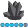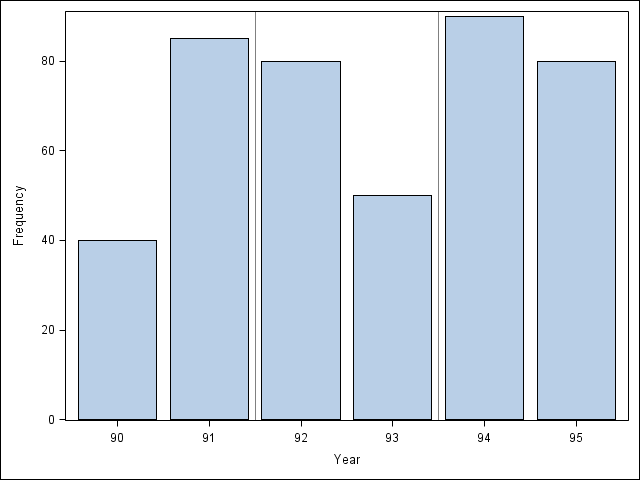paolo_santin
Calcite | Level 5

## Vertical reference lines on proc gchart

Hi!

I would like to include some vertical reference lines inside a proc gchart graph.

Is there anyway to use the tags vref instead of using a annotate dataset?

/paolo

goptions reset=all border cback=white htitle=12pt;

/* Create input data set, A */
data a;
input Year Y;
datalines;
90 40
91 85
92 80
93 50
94 90
95 80
;
run;

/* Create the Annotate data set */
data anno_ds;
length color function \$8 text \$14;
retain xsys '1' ysys '2' when 'a';
function='move'; x=33.5; y=0; output;
function='draw'; x=33.5; y=100; color='black'; size=2; output;
function='move'; x=67; y=0; output;
function='draw'; x=67; y=100; color='black'; size=2; output;
run;

/* Add a title to the graph */
title1 'Sales Report';

/* Define axis characteristics */
axis1 order=(0 to 100 by 25);

/* Produce the bar chart using the ANNO= */
/* option on the VBAR statement */
proc gchart data=a;
vbar year / sumvar=y raxis=axis1 discrete
width=8 anno=anno_ds;
run;
quit;1 ACCEPTED SOLUTION

Accepted SolutionsAncaTilea
Pyrite | Level 9

## Re: Vertical reference lines on proc gchart

On a second thought, if you have access to SAS 9.2. +  then you could use the SGPLOT

proc sgplot data=a;

vbar year /freq = y ;

refline 91 93 / axis = x discreteoffset = 0.5;

/*axis = x tells SAS this is a vertical line */

/*the discreteoffset tells SAS to move to line 0.5 units (to the right) from the 91 and 93 - thus giving you 91.5, and 93.5)*/

run;

I am fairly new to SGPLOT, but it turns out it has lots of features ready to be used.

Best of luck, again!4 REPLIES 4AncaTilea
Pyrite | Level 9

## Re: Vertical reference lines on proc gchart

Hi.

Unfortunately, PROC GCHART of SAS does not allow for a "vref = " option. (It allows it, but it doesn't produce anything).

The best way to go about drawing vertical lines on a bar-plot is to use the annotate capability.

(Of course, you could also tinker around with footnotes and move/draw combination but it's not worth it if you have the annotate in place).

Best of luck!AncaTilea
Pyrite | Level 9

## Re: Vertical reference lines on proc gchart

On a second thought, if you have access to SAS 9.2. +  then you could use the SGPLOT

proc sgplot data=a;

vbar year /freq = y ;

refline 91 93 / axis = x discreteoffset = 0.5;

/*axis = x tells SAS this is a vertical line */

/*the discreteoffset tells SAS to move to line 0.5 units (to the right) from the 91 and 93 - thus giving you 91.5, and 93.5)*/

run;

I am fairly new to SGPLOT, but it turns out it has lots of features ready to be used.

Best of luck, again!paolo_santin
Calcite | Level 5

## Re: Vertical reference lines on proc gchart

Thank you very much!

## Re: Vertical reference lines on proc gchart

Correct - the only way (I know of) to draw reference lines between the bars in Gchart is to annotate them.

One work-around is to use Gplot, with the 'needle' interpolation, and make the needles very wide so they look like bars.  One advantage here is that you will be able to use x-values directly to position the reference lines (such as 91.5, rather than having to calculate that it's at position x=33.5%).

A Gplot line chart is not really a bar chart, so you have a few trade-offs ... for example, the bars will be spaced out proportionally rather than evenly-spaced (so hopefully your x-values are always evenly-spaced increments), and since these are 'lines' and not bars you will hot be able to associate html hover-text or drilldowns with them.

That being said, here is the code:

title1 'Sales Report';

axis1 order=(0 to 100 by 25) minor=none offset=(0,0);

axis2 minor=none offset=(10,10);

symbol1 value=none interpol=needle width=40;

proc gplot data=a;

plot y*year=1 / vaxis=axis1 haxis=axis2

href=91.5 93.5;

run;

Discussion stats
• 4 replies
• 5427 views
• 6 likes
• 3 in conversation